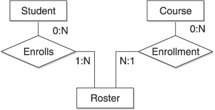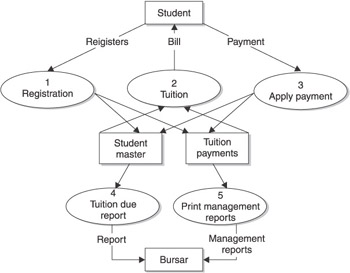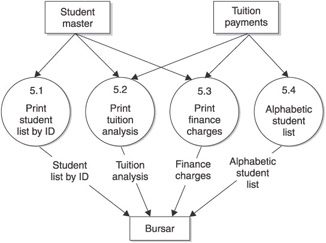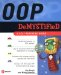# Relationships Among Objects

Some objects have functional relationships rather than a hierarchical relationship. A functional relationship is one where two or more objects interact with each other but are not directly related to each other. A hierarchical relationship is one where objects are directly related through inheritance.

For example, a student fills out a registration form in order to register for a course. The student and the registration form are both objects that have a functional relationship, and neither is directly related through inheritance. The functional relationship is that a student uses a registration form to register for class.

Object-oriented programmers determine the functional relationship among objects in order to help them understand how objects work together to achieve the goal of the application.

An object is sometimes referred to as an entity . For example, a student is an entity, the registration form is an entity, and a course is an entity. A functional relationship is also known as an entity relationship .

Programmers categorize entity relationships in four ways:

### One to One

A one-to-one entity relationship is where one entity is related to only one instance of another entity, such as one student has one dorm room.

### One to Many

A one-to-many entity relationship is where one entity is related to many instances of another entity, such as one student takes multiple courses.

### Many to One

A many-to-one entity relationship is where many instances of an entity are related to one instance of another entity, such as many students are assigned to one course.

### Many to Many

A many-to-many entity relationship is where many instances of an entity are related to many instances of another entity, such as many students assigned to many classrooms.

### Entity Relationship Diagram

Programmers illustrate entity relationships in an entity relationship diagram. An entity relationship diagram depicts a graphic representation of how one entity is associated with another entity.

An entity relationship diagram contains three components: entities, the functional relationship, and a ratio that indicates the type of relationship. Look at Figure 7-4 to see how these components are used.Figure 7-4: tionship diagram of a student entity and a course entity

Figure 7-4 is an entity relationship between a student and a course. The functional relationship is that a student enrolls in a course, and a course has enrollment. Both of these functions result in a roster for the course. The roster is also an entity.

The type of a relationship is represented by a ratio. The student ratio 0:N means that a student can register for no courses or many courses. The N in the ratio implies any number. That is, a student can still be a student at the college without having to register for a course. The course ratio 0:N means that a course might have no students enrolled or many students enrolled. That is, the college can offer a course and no one enrolls in the course.

The ratio changes once a student registers for a course to reflect a change in relationship among the entities. The student ratio is now 1:N, meaning that the student registered for at least one course, but could register for many courses. Likewise, the course ratio is N:1, indicating that many students can enroll in one course.

Programmers use an entity relationship diagram to help in the design of their application so as to ensure that the application can handle all possible relationships. For example, the registration application must be able to handle a course without an enrollment and a course where any number of students can be enrolled.

### Leveling Diagram

An object-oriented application can easily become complex and difficult to understand. Programmers simplify this complexity by using a leveling diagram, which depicts an application in layers , where the top level has the least amount of detail and is the easiest to understand. Subsequent levels have more detail and are usually the hardest to understand.

Each level illustrates entities and processes. As the programmer drills down into the leveling diagram, they learn more details about each process. Figure 7-5 shows the first level of a leveling diagram that depicts how the bursar collects tuition from students.Figure 7-5: The first level of the leveling diagram shows all the processes involved in how the bursar collects tuition from students.

Rectangles are used to represent entities, which are Student and Bursar in this example. Circles represent processes, such as registration, tuition, and payment of tuition. Also, two parallel lines are used to signify a data store, which is simply a place where data is stored. This example has two data stores: one that stores student information and another that stores tuition information. Arrows are used to show a movement of data among entities, processes, and data stores.

Notice that each process is assigned a unique number. This number is used to connect the first level with lower levels of the leveling diagram. Take a look at Figure 7-6 and you ll see how this works.Figure 7-6: Level 2 of the leveling diagram shows details of the Print Management Reports process from Level 1.

Figure 7-6 is the second level of the level diagram and describes the Print Management Reports process shown on Level 1. The Print Management Reports process actually has many subprocesses ”one for each report. The second level shows these processes. Notice that each of the subprocesses is assigned a unique number. The number begins with corresponding number of the process shown on Level 1. Each of these subprocesses begins with 5, which is the number of the Print Management Reports process in Level 1.

The subprocess number contains a decimal followed by another number, which represents the subprocess in Level 2. For example, Print Student List By ID is the first subprocess on Level 2 and therefore is numbered 5.1. The number 5 links this subprocess to a process on Level 1, and the number 1 indicates this is the first subprocess on Level 2.

The same progression is used for subsequent levels. For example, you would look in Level 3 at process 5.1.1 to learn the details of the process used to print the student list by ID.OOP Demystified
ISBN: 0072253630
EAN: 2147483647
Year: 2006
Pages: 130

Similar book on Amazon